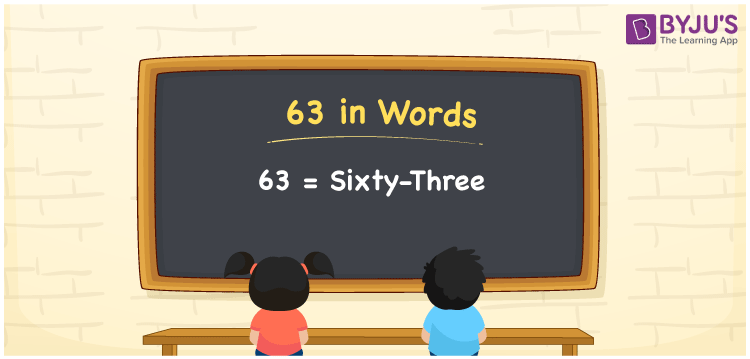# 63 in Words

63 in Words is written as “Sixty-three”. In Maths, 63 is a number that represents a count or a value. When it is expressed as a count, it is a counting number or a cardinal number. For example, there are Sixty-three candies in a jar. In English, the spelling of 63 is Sixty-three. It is used to represent a value such as 63 rupees in words, written as Sixty-three Rupees or Rupees Sixty-three. Learn how to write the number 63 in words in this article. You can write numbers in words using the English alphabet. You can read 63 as “Sixty-three” in English.

 63 in words Sixty-three Sixty-three in Numbers 63

## 63 in English Words## How to Write 63 in Words?

The topic of writing 63 in words using the place value chart can be learnt in this section. The detailed explanation given here helps students get a good hold on the basic concepts of counting numbers. 63 is a two digit number and the place value chart for the number is provided in a table form here.

 Tens Ones 6 3

63 in expanded form is discussed here:

6 × Ten + 3 × One

= 6 × 10 + 3 × 1

= 60 + 3

= 63

= Sixty-three

Therefore, 63 in words is written as Sixty-three.

63 is a natural number that precedes 64 and succeeds 62.

63 in words – Sixty-three

Is 63 an odd number? – Yes

Is 63 an even number? – No

Is 63 a perfect square number? – No

Is 63 a perfect cube number? – No

Is 63 a prime number? – No

Is 63 a composite number? – Yes

## Frequently Asked Questions on 63 in Words

Q1

### How can the number 63 be written in words?

63 can be written in words as “Sixty-three”.
Q2

### How to write 63 on a cheque?

63 can be written on a cheque as Sixty-three rupees only.
Q3

### Is 63 an odd number?

63 is an odd number as it cannot be divided by 2.